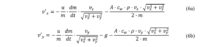# Numerical methods: relevance

#### Fiidweee

##### New member
This question is about why I'm requested by my teacher to employ numerical methods instead of analytical ones.

The subject I'm writing about is rocket science. The task is to predict the movement of a rocket in 2 dimensions. I understand this is a maths forum, but we'll get there.
I'm able to construct two acceleration equations:u, g, A, cw, p (rho), dm/dt are all constants while m (mass of the rocket) and vy and vx change depending on the time (speed in vertical and horizontal direction respectively). Since I'm interested in the position functions, I must solve second order differential equations.

I wrote in my project that they are difficult to solve analytically because it's a 'linked/codependent equation system' without really knowing what I was talking about. Basically, I need to understand why it's relevant to use numerical methods instead of analytical ones in this problem.

#### HallsofIvy

##### Elite Member
You say it is difficult to use analytical methods- how difficult would numerical methods be?

#### Fiidweee

##### New member
You say it is difficult to use analytical methods- how difficult would numerical methods be?
I don't know if it is difficult to use analytical methods, I guess that's what I'm trying to figure out.

As a high school student, it was rather difficult to manage.
To carry out the task, I've written a program that manages the large amount of calculational loops. Each step depends on the previous one.
1. The acceleration is calculated in each direction with the equations above.
2. The velocity is calculated with the help of Runge-Kutta 4th order.
3. The position is calculated with the help of forward Euler.

Any help is greatly appreciated as I truly need to try to understand. It was quite a route to take, so why not do it analytically. I don't know how you would do it analytically nonetheless.

#### JeffM

##### Elite Member
First, many differential equations are not currently solvable using analytic methods. The only way to "solve" them so far is approximately by numerical methods.

Second, it may be less work to write a program than to try to find an analytic solution. (Remember that there may not be one to find, and even if there is one, who knows how long it might take to find it.)

#### Fiidweee

##### New member
First, many differential equations are not currently solvable using analytic methods. The only way to "solve" them so far is approximately by numerical methods.

Second, it may be less work to write a program than to try to find an analytic solution. (Remember that there may not be one to find, and even if there is one, who knows how long it might take to find it.)

That depends, is it unsolvable in this case? If I am questioned at the examination, I'll need to be able to answer for this very specific case.

I understand that some differential equations are unsolvable, if not most, but I'm not sure if I'm to say that this would be too difficult for me to manage, or that it is indeed impossible. Saying something is impossible is quite 'extreme' so to speak.

#### topsquark

##### Full Member
The system of equations is pretty non-linear. That's not to say that analytical solutions don't exist but unless you are a masochist I agree that your best bet is a numerical solution.

-Dan

•yoscar04 and Subhotosh Khan

#### Subhotosh Khan

##### Super Moderator
Staff member
The system of equations is pretty non-linear. That's not to say that analytical solutions don't exist but unless you are a masochist I agree that your best bet is a numerical solution.

-Dan
I don't know about that! Beauty - they say, is in the eye of the beholder.

•topsquark

#### Fiidweee

##### New member
So as far as my high school maths are able to contribute to my understanding of what you guys are writing, it's not easily discerned if it's analytically solvable. And if it is, it would take a large amount of time to figure out.

How would one explain why this is? What properties/characteristics of the equations make them difficult to solve analytically? I'm afraid I'll need that spelled out before I really catch on.

#### Subhotosh Khan

##### Super Moderator
Staff member
So as far as my high school maths are able to contribute to my understanding of what you guys are writing, it's not easily discerned if it's analytically solvable. And if it is, it would take a large amount of time to figure out.

How would one explain why this is? What properties/characteristics of the equations make them difficult to solve analytically? I'm afraid I'll need that spelled out before I really catch on.
If you do a google search using:

methods of solving non-linear differential equations

You will get 14*107 "hits".